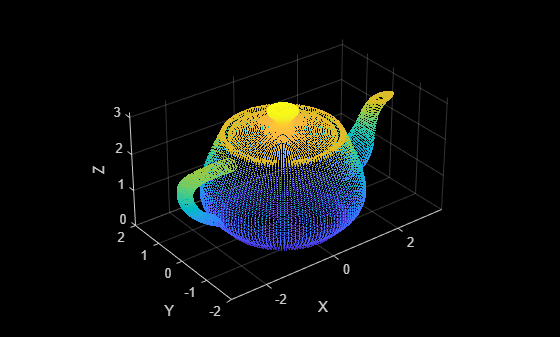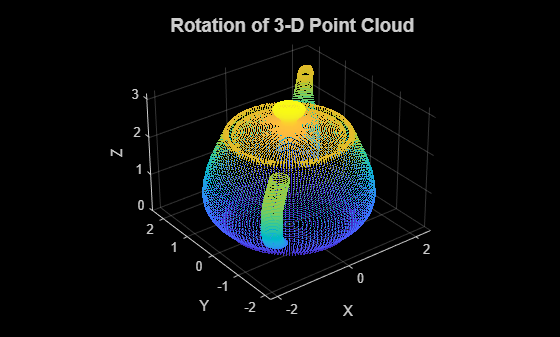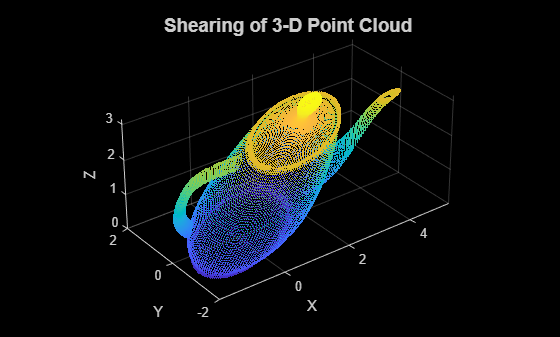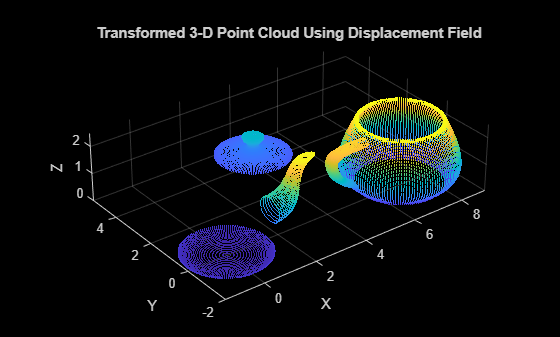# pctransform

Transform 3-D point cloud

## Syntax

``ptCloudOut = pctransform(ptCloudIn,tform)``
``ptCloudOut = pctransform(ptCloudIn,D)``

## Description

example

````ptCloudOut = pctransform(ptCloudIn,tform)` applies the specified 3-D affine transform, `tform` to the point cloud, `ptCloudIn`. The transformation can be a rigid, similarity, or affine transformation.```

example

````ptCloudOut = pctransform(ptCloudIn,D)` applies the displacement field `D` to the point cloud. Point cloud transformation using a displacement field define translations with respect to each point in the point cloud.```

## Examples

collapse all

`ptCloud = pcread('teapot.ply');`

Plot the point cloud.

```figure pcshow(ptCloud) xlabel('X') ylabel('Y') zlabel('Z')```Create a transformation object with a 45 degree rotation along the z-axis.

```rotationAngles = [0 0 45]; translation = [0 0 0]; tform = rigidtform3d(rotationAngles,translation);```

Transform the point cloud.

`ptCloudOut = pctransform(ptCloud,tform);`

Plot the transformed point cloud.

```figure pcshow(ptCloudOut) xlabel('X') ylabel('Y') zlabel('Z')```This example applies a rigid transformation (rotation) and a nonrigid transformation (shear) to a 3-D point cloud.

Read a point cloud into the workspace.

`ptCloud = pcread('teapot.ply');`

Plot the original 3-D point cloud.

```figure1 = figure; axes1 = axes(Parent=figure1); pcshow(ptCloud,Parent=axes1,AxesVisibility='on'); xlabel('X'); ylabel('Y'); zlabel('Z'); title('3-D Point Cloud',FontSize=14)```Rotation of 3-D Point Cloud

Create a rigid transformation object that defines a 45 degree rotation along the z-axis.

```rotationAngles = [0 0 45]; translation = [0 0 0]; tform = rigidtform3d(rotationAngles,translation);```

Transform the point cloud.

`ptCloudOut1 = pctransform(ptCloud,tform);`

Plot the rotated point cloud.

```figure2 = figure; axes2 = axes(Parent=figure2); pcshow(ptCloudOut1,Parent=axes2,AxesVisibility='on'); xlabel('X'); ylabel('Y'); zlabel('Z'); title({'Rotation of 3-D Point Cloud'},FontSize=14)```Shearing of 3-D point cloud

Create an affine transformation object that defines shearing along the x-axis.

```A = [1 0.75 0.75 0; ... 0 1 0 0; ... 0 0 1 0; ... 0 0 0 1]; tform = affinetform3d(A);```

Transform the point cloud.

`ptCloudOut2 = pctransform(ptCloud,tform);`

Plot the transformed point cloud.

```figure3 = figure; axes3 = axes(Parent=figure3); pcshow(ptCloudOut2,Parent=axes3,AxesVisibility='on'); xlabel('X'); ylabel('Y'); zlabel('Z'); title({'Shearing of 3-D Point Cloud'},FontSize=14)```Read a point cloud into the workspace.

`ptCloud = pcread('teapot.ply');`

Create a displacement field `D` of same size as the point cloud.

`D = zeros(size(ptCloud.Location));`

Set the displacement field value along x-axis for the first half of the points to 7.

```pthalf = ptCloud.Count/2; D(1:pthalf,1) = 7;```

Extract the indices of points within a region-of-interest (ROI) using the `pointCloud` method `findNeighborsInRadius`. Set the displacement field value along the x-, y-, and z-axis for points within the ROI to 4, 4, and -2, respectively.

```indices = findNeighborsInRadius(ptCloud,[0 0 3.1],1.5); D(indices,1:2) = 4; D(indices,3) = -2;```

Transform the point cloud using the displacement field.

`ptCloudOut = pctransform(ptCloud,D);`

Display the original and transformed point cloud.

```figure pcshow(ptCloud) xlabel('X'); ylabel('Y'); zlabel('Z'); title('Original 3-D Point Cloud')``````figure pcshow(ptCloudOut) xlabel('X'); ylabel('Y'); zlabel('Z'); title('Transformed 3-D Point Cloud Using Displacement Field')```## Input Arguments

collapse all

Point cloud, specified as a `pointCloud` object.

3-D geometric transformation, specified as a `rigidtform3d`, `simtform3d`, or `affinetform3d` object. See Specify Transformation Matrix for details on how to set up an affine 3-D `tform` input.

Displacement field, specified as either M-by-3 or an M-by-N-by-3 array. The displacement field is a set of displacement vectors that specify the magnitude and direction of translation for each point in the point cloud. The size of the displacement field must be the same as the size of the `Location` property of the `pointCloud` object.

Data Types: `single` | `double`

## Output Arguments

collapse all

Transformed point cloud, returned as a `pointCloud` object. The transformation applies to the coordinates of points and their normal vectors.

## Version History

Introduced in R2015a

expand all